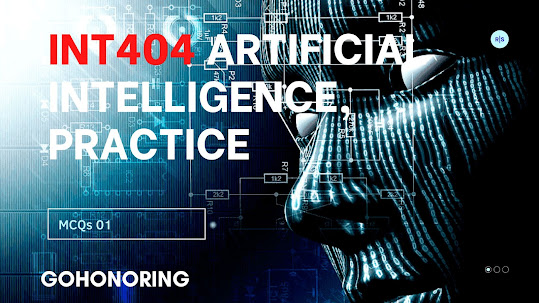### Ticker

6/recent/ticker-posts

# INT404 Artificial Intelligence, Practice MCQs 02

INT404 Practice MCQs 02, 15 Questions.

1. Knowledge representation is represented in two ways in AI

• a. machine logic
• b. predicate logic
• c. propositional logic
• d. compund logic

2. Generate the Soundex code of “VALIDATION”

• a. V433
• b. V435
• c.V432
• d.V434

3. Transform word “MAXIMIZE” into “MINIMIZE”, then how many edit operations required.

• a. 2 substitution
• b. 1 insertion, 1 substitution
• c. 1 deletion , 1 insertion
• d. 2 insertion

4. To work with Natural Language Toolkit , the first step is to install package, which is the right command to install the package.

• a. pip install nltk
• b. install nltk
• c. pip install
• d. pip nltk

5. Transform word “HERE” into “HEAR”, then how many edit operations required.

• a. 2 substitution
• b. 2 insertion
• c. 1 insertion and 1 substitution
• d. 2 substitution and 1 insertion

6. Error caused by extra element is due to :

• a. insertion
• b. substitution
• c. deletion
• d. both a and b

7. While constructing the parse tree, the base proposition would be:

• a. S
• b. VP
• c. NP
• d. T

8. In NLP, sentence meaning is represented at

• a. syntactic phase
• b. semantic phase
• c. discourse integration
• d. none.

9. Grammatical errors checking done by

• a. semantic analysis
• b. syntactic analysis
• c. both a and b
• d. pragmatic analysis

10. Bag of Words (BoW) model is a simple algorithm used in Natural Language Processing for…

• a. feature extraction
• b. feature selection
• c. pre-processing
• d. none

11. Bag of word models doesn’t respect the semantics of the word.

• a. true
• b. false

12. Which method is imported from NLTK package to generate Lemma.

• a. wordnetlemmatizer
• b. WordNetLemmatizer
• c. NetLemmatizer
• d. WordnetLemmatizer

13. If lemmatization is used, the base form of word “Talking” is..

• a. Tal
• b. Talk
• c. Talki
• d. Talkin

14. To construct the complex sentences, we required

• a. connectives
• b. logical connectives
• c. symbols
• d. all of the above

15. In propositional logic how many symbols are there?

• a. 2
• b. 3
• c. 4
• d. 1INT404 Artificial Intelligence, Practice MCQs 01.  Click Hera